# Plus Two Chemistry Chapter Wise Questions and Answers Chapter 2 Solutions

Students can Download Chapter 2 Solutions Questions and Answers, Plus Two Chemistry Chapter Wise Questions and Answers helps you to revise the complete Kerala State Syllabus and score more marks in your examinations.

## Kerala Plus Two Chemistry Chapter Wise Questions and Answers Chapter 2 Solutions

### Plus Two Chemistry Solutions One Mark Questions and Answers

Question 1.
Which of the following is a liquid in solid type solution?
(a) glucose dissolved in water
(b) Camphor in N2
(c) amalgam of Hg with Na
(d) Cu dissolved in Au
(c) amalgam of Hg with Na

Question 2.
The concentration term used when the solute is present in trace quantities is _______
ppm (parts per million)

Question 3.
A binary solution of ethanol and n-heptane is an example of
(a) ideal solution
(b) Non-ideal solution with +ve deviation
(c) Non-ideal solution with -ve deviation
(d) unpredictable behaviour
(b) Non-ideal solution with +ve deviationQuestion 4.
A solution which has higher osmotic pressure as compared to other solution is known as _____
Hypertonic

Question 5.
Which of the following solutions will have the highest boiling point at 1 atm pressure?
(a) 0.1M FeCl3
(b) 0.1MBaCl2
(c) 0.1MNaCl
(d) 0.1Murea
(a) 0.1M FeCl3

Question 6.
1 kilogram of sea water sample contains 6 mg of dissolved O2. The concentration of O2 in the sample in ppm is
6.0

Question 7.
The amount of solute (molar mass 60 g mol-1) that must be added to 180 g of water so that the vapour pressure of water is lowered by 10% is
60 gPure Aqua provides users with an osmotic pressure calculator to gain better insight on your osmotic pressure requirements.

Question 8.
The correct equation for the degree of association (a) of an associating solute ‘n‘ molecules of which undergoes association in solution is
a = $$\frac{n(i-1)}{1-n}$$

Question 9.
A solution is prepared by dissolving 10 g NaOH in 1250 ml of a solvent of density 0.8 ml/g. The molarity of the solution is _______
0.25

Question 10.
If the elevation in boiling point of a solution of non volatile, non electrolyte in a solvent (Kb = xk. kg mol-1) is 7 K, then the depression in freezing point would be kf = ZK kg mol-1
$$\frac{Y Z}{x}$$

### Plus Two Chemistry Solutions Two Mark Questions and Answers

Question 1.
Match the terms of list A with those in list B.

 A B Raoult’s Law. Colligative property. Henry’s Law. Ideal solution. Elevation of boiling point. Solution of gases in liquids. Benzene + Toluene. Vapour pressure of solutions.

 A B Raoult’s Law. Vapour pressure of solutions. Henry’s Law. Solution of gases in liquids. Elevation of boiling point. Colligative property. Benzene + Toluene. Ideal solution.Question 2.
At a particular temperature, the vapour pressure of two liquids A and B are 120 and 180 mm of Hg respectively. Two moles of A and 3 Moles of B are mixed to form an ideal solution. What is the vapour pressure for the solution?
A = 120mm of Hg
B= 180 mm of Hg
χA = 2/5
χB =3/5
PA = P°A × χA
= 120 × 2/5 = 48 mm of Hg
PB = P°B × χB
= 180 × 3/5 = 108 mm of Hg
Ps = PA + PB
= 108 + 48 = 156 mm of Hg

Question 3.
Find the volume of H2O that should be added to 300 mL of 0.5 M NaOH so as to prepare a solution of 0.2 M.
M1V1 = M2V2
300 × 0.5 = V2 × 0.2
V2 $$\frac{300 \times 5}{2}$$ = 750 mL
H2O to be added = 750 mL – 300 mL = 450mL

Question 4.
Calculate the osmotic pressure of 5% solution of urea at 27°C?
Mass of urea, WB = 5 g
R = 0.0821 L atm K-1 mol-1
T = 273 + 27°C = 300 K
Molecular mass of urea, MB = 60 g mol-1
V = 100 mL = 0.1L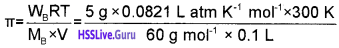= 20.53 atmQuestion 5.
Osmotic pressure of 1M solution of NaCl is approximately double than that of 1M sugar solution. Why?
Osmotic pressure is a colligative property and it depends on the number of solute particles present in the solution. In solution, each NaCl unit undergoes dissociation to form two particles (NaCl → Na+ + Cl) and hence osmotic pressure of 1M NaCl is twice that of 1M sugar solution. Sugar molecules does not undergo association or dissociation in solution.

Question 6.
What do you mean by ideal solution?
Ideal solution is a solution which obeys Raoult’s law over the entire range of concentration and temperature, i.e., for an ideal solution having two volatile components A and B.
PA = P°A χA, PB = P°B χB,
Ps = PA + PB =P°A χA + P°B χB

Question 7.
Many countries use desalination plants to meet their potable water requirements. Comment on the phenomenon behind it.
This is based on reverse osmosis. When a pressure more than osmotic pressure is applied, pure water is squezed out of the sea water through a semi-permeableQuestion 8.
Movement of solvent molecules through a semipermeable membrane from pure solvent to the solution side is called osmosis.
What are the following

1. Isotonic solution
2. Hypertonic solution?

1. Isotonic solution:
If the osmotic pressure of the two solutions are equal, they are called isotonic solutions.

2. Hypertonic solution:
A solution having higher osmotic pressure than another solution is called hypertonic solution.Question 9.
What is meant by azeotrope?
Liquid mixtures which distil without change in composition are called azeotropic mixtures or azeotropes.

### Plus Two Chemistry Solutions Three Mark Questions and Answers

Question 1.
A student was asked to define molality. Then he answered that it is the number of gram moles of the solute dissolved per litre of the solution.

1. Is it correct?
2. Can you help the student to define molality?
3. Calculate the molality of a solution containing 20 g of NaOH in 250 g of H2O.

1. It is not correct.
2. It is the number of moles of the solute present in 1000 g of the solvent. Molality can be determined by using the formula.
Molality, m = $$\frac{\text { Mass of the solute in gram } \times 1000}{\text { Molar mass of the solute } \times \text { Mass of the solvent in gram }}$$
3. Molality, m = $$\frac{20 \times 1000}{40 \times 250}$$ = 2 m

Question 2.
The graph of non-ideal solution showing -ve deviation as drawn by a student is given below:1. Is this diagram correct?
2. Substantiate your argument with a suitable example.

1. No.
2. Because this graph shows the non-ideal solution showing +ve deviation and not -ve deviation. Consider a solution obtained by mixing chloroform and acetone. Here chloroform molecule is able to form hydrogen bond with acetone molecule as shown below: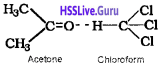As a result of this, vapour pressure of the solution decreases. Due to this, boiling point increases. The volume of the solution is less than the expected value. The mixing process is exothermic. So the actual graph is as given below: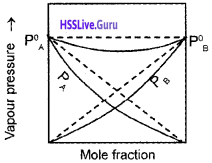Question 3.
Some words are missed in the following paragraph. Add suitable words in the blanks:
If osmotic pressure of 2 solutions are equal they are called ______(a)_____ solution. The solution which is having ______(b)______ osmotic pressure is called hypertonic solution and the solution which is having lower osmotic pressure is called ______(c)_____ solution.

1. Isotonic
2. Higher
3. HypotonicQuestion 4.
The solubility of gases depends upon some factors.

1. Can you suggest the factors?
2. Which is the law behind it?
3. What are the limitations of this law?

1. Nature of the gas, Nature of the solvent, Pressure, Temperature
2. Henry’s law. It states that the mass of a gas dissolved per unit volume of the solvent at a given temperature is proportional to the pressure applied on it.
3. Henry’s law is valid only under the following conditions:
• The pressure of the gas is not too high.
• The temperature is not too low.
• The gas is not highly soluble.

Question 5.
A solution of 12.5 g of an organic solute in 170g of H2O a boiling point elevation of 0.63 K. Calculate the molecular mass of the solute (K2=0.52 K/m).
Kb for water = 0.52 K Kg mol-1
WA = 170g
ΔTb = 0.63 K
WB = 12.5 g
MB = ?Question 6.
Find the freezing point of the solution containing 3.6 g of glucose dissolved in 50 g of H20. (Kf for H2O = 1.86 K/m).
Mass of glucose, WB = 3.6 g
Molecular mass of glucose = 180 g mol-1
Mass of solvent, WA = 50 g
Kf for H2O = 1.86 K/m = 1.86 K kg-1 mol-1i.e., ΔTf = T°f – Tf= 0.744 K
∴ Freezing point of the solution, Tf = T°f – ΔT
= 273 K – 0.744 K = 272.3 K

Question 7.
Raw mangoes shrivel when pickled in brine solution.

1. Name the process behind this.
2. Define that process.

1. Osmosis
2. When a solution is separated from its solvent by a semipermeable membrane, the solvent flows into the solution through the semipermeable membrane. This process is called osmosis.Question 8.
200 cm3 of an aqueous of a protein contains 1.26 g of the protein. The osmotic pressure of such a solution at 300 K is found to be 2.57 × 10-3 bar. Calculate the molar mass of the protein.Question 9.
What type of deviation from Rauolt’s law is exhibited by a mixture of phenol and aniline? Explain with the help of graph.
Negative deviation.In this case the intermolecular hydrogen bonding between phenolic proton and lone pair on nitrogen atom of aniline is stronger than the respective intermolecular hydrogen bonding between similar molecules. Thus, escaping tendency of the particles decreases in solution and hence the liquid mixture shows negative deviation.

Question 10.
Wilted flowers revive when placed in fresh water.

1. Identify and define the phenomenon.
2. Mixing of acetone and CHCl3 results in a reduction in volume. What type of deviation from Raoult’s law is observed here? Give reason.
3. Benzoic acid dissolved in benzene shows double of its molecular mass. Explain.

1. Osmosis. It is the phenomenon of flow of solvent from pure solvent into a solution or from a solution of lower concentration into a solution of higher concentration through a semi-permeable membrane.

2. Negative deviation. Here chloroform molecule is able to form hydrogen bond with acetone molecule.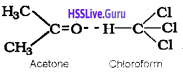Thus escaping tendency of the particles decreases in solution and hence the liquid mixture shows negative deviation.

3. Benzoic acid undergoes association in solution.
2C6H5COOH $$\rightleftharpoons$$ (C6H5COOH)2 Thus, the number of particles as well as colligative properties decreases. So molecular mass increases.

Question 11.
A solution is obtained by mixing 300 g of 25 % solution and 400 g of 40 % solution by mass. Calculate the mass percentage of the resulting solution.
Mass of solute in 300 g of 25 % solution
$$\frac{300 \times 25}{100}$$ = 75 g
Mass of solute in 400 g of 40 % solution
$$\frac{400 \times 40}{100}$$ = 160 g
Total mass of solute = (75 + 160) g = 235 g
Total mass of solution = (300 + 400) g = 700 g
Mass % of solute in resulting solution = $$\frac{235 \times 100}{700}$$ = 33.57%
Mass % of solvent (water) in resulting solution
= 100 – 33.57 = 66.43%

Question 12.
A graph showing vapour pressure against mole fraction of an ideal solution with volatile components A and B are shown below:1. Which law is supported by the graph?
2. State the law.
3. Resketch the graph if the attraction between A and B is greater than that between A-A and B-B.

1. Raoult’s law.
2. Raoult’s law states that vapour pressure of a volatile component in a solution is the product of vapour pressure of that component in the pure form and mole fraction of that component in the solution.
3. If the A-B attraction is greater than A-A and B-B attractions the liquid mixture behaves as a non-ideal solution with negative deviation.Question 13.
Concentrated nitric acid used in the laboratory work is 68% nitric by mass in aqueous solution. What should be the molarity of such a sample of the acid if the density of the solution is 1.504 g mL-1?Question 14.
Why does gases always tend to be less solube in liquids as the temperature is raised?
Dissolution of gases is exothermic process. It is because of the fact this process involves decrease of entropy (ΔS < 0). Thus, increase of temperature tends to push the equilibrium,
Gas + Solvent $$\rightleftharpoons$$ Solution; ΔH = -ve
in the backward direction, thereby, supressing the dissolution.

Question 15.
The vapour pressure of water is 12.3 kPa at 300 K. Calculate vapour pressure of 1 molal solution of a non-volatile solute in it.
H2O= 12.3 kPa
1000
In 1 molal solution, nsolute = 1; nH2O= $$\frac{1000}{18}$$ = 55.5
∴ χH2O = $$\frac{55.5}{55.5+1}$$
Vapour pressure of the solution, Ps = P°H2O × χH2O
= 0.982 × 12.3 = 12.08 kPa

Question 16.
Determine the osmotic pressure of a solution prepared by dissolving 25 mg of K2SO4 in 2 litre of water at 25°C, assuming that it is completely dissociated.
Since K2SO4 is completely dissociated as K2SO4 → 2K+ + SO42- Thus, i = 3
Osmotic pressure of the solution, π = i CRT
$$\frac{3 \times 25 \times 10^{-3} \mathrm{g} \times 0.0821 \mathrm{L} \mathrm{atm} \mathrm{K}^{-1} \mathrm{mol}^{-1} \times 298.15 \mathrm{K}}{174 \mathrm{g} \mathrm{mol}^{-1} \times 2 \mathrm{L}}$$
= 5.27 × 10-3 atm

Question 17.
Solution of sucrose is prepared by dissolving 34.2 g of it in 1000 g of water. Find out the freezing point of the solution, if Kf of water is 1.86 K/kg/mol? (Molecular mass of sucrose is 342 g/mol).
ΔTf = kf × m
=1.86 K kg mol-1 × $$\frac{34.2 \mathrm{g} \times 1000 \mathrm{g} \mathrm{kg}^{-1}}{342 \mathrm{g} \mathrm{mol}^{-1} \times 1000 \mathrm{g}}$$ = 0.186K
Tf = OK – 0.186 K
Freezing point of the solution = – 0.186 KQuestion 18.
At 300 K, 36 g of glucose present in a litre of its solution has an osmotic pressure of 4.98 bar. If the osmotic pressure of the solution is 1.52 bars at the same temperature, what would be its concentration?
In the first case, π1 = C1RT
i.e., 4.98 bar = $$\frac{36 \mathrm{g} \times \mathrm{R} \times 300 \mathrm{K}}{180 \mathrm{g} \mathrm{mol}^{-1}}$$ ——– (1)
In second case, π2 = C2RT
i.e., 1.52 bar = C2R × 300 K ———- (2)Question 19.
A solution containing 12.5 g of non-electrolytic substance in 175 g of water gave boiling point elevation of 0.70 K. Calculate the molecular mass of the substance? (Kb for water = 0.52 K kg mol-1)
Molecular mass of the solute, MB = $$\frac{1000 \mathrm{K}_{\mathrm{b}} \mathrm{W}_{\mathrm{B}}}{\mathrm{W}_{\mathrm{A}} \Delta \mathrm{T}_{\mathrm{b}}}$$
$$\frac{1000 \mathrm{g} \mathrm{kg}^{-1} \times 0.52 \mathrm{K} \mathrm{kg}^{-1} \mathrm{mol}^{-1} \times 12.5 \mathrm{g}}{175 \mathrm{g} \times 0.70 \mathrm{K}}$$
= 53.06 mol-1

Question 20.

1. “For any solution the partial vapour pressure of each volatile component in the solution is directly proportional to its mole fraction. Name the law stated above.
2. Study the graph. What phenomenon it denotes? Based on your observation predict the reason for the greater volatility of a mixture of carbon disulphide and acetone?1. Raoult’slaw
2. Positive deviation from Raoult’s law. In a solution formed by adding CS2 to acetone, the dipolar interactions between solute-solvent molecules are weaker than the respective interactions among the solute-solute and solvent-solvent molecules. Thus, the escaping tendency of the particles increases and the solution shows +ve deviation.

Question 21.

1. To get hard boiled eggs, common salt is added to water during boiling. Give reason.
2. Which colligative property is more suitable for the determination of molecular mass of polymers? Give the expression to determine molecular mass by this method.

1. The egg which is placed in sodium chloride solution will shrink due to osmosis of water out of the egg and thus get hardened.
2. Osmotic pressure method.

Question 22.
18 g of glucose is dissolved in 1kg of water in a beaker. At what temperature will water boil at 1.013 bar? (Kb for water is 0.52 K kg mol-1)
Number of moles of glucose = 18/180 = 0.1 mol.
Mass of solvent = 1 kg.
Morality of glucose , m = $$\frac{n_{8}}{W_{A}}$$
= $$\frac{0.1 \mathrm{mol}}{1 \mathrm{kg}}$$ = 0.1 mol/Kg
Elevation of boiling point ΔTb = Kb × m
= 0.52 K kg/mol × 0.1 mol/kg = 0.052 K
Since water boils at 373.15 K at 1.013 bar pressure, the boiling point of solution will be 373.15 K + 0.052 K = 373.202 K

### Plus Two Chemistry Solutions Four Mark Questions and Answers

Question 1.
Colligative properties are exhibited by dilute solutions.

1. What do you mean by colligative properties?
2. Which are the four colligative properties?

1. Colligative properties are those properties of dilute solutions of non-volatile solutes whose value depend upon the number of solute particles irrespective of their nature relative to the total number of particles present in the solution.
2. The four colligative properties
• Relative lowering of vapour pressure
• Elevation of boiling point
• Depression of freezing point
• Osmotic pressureQuestion 2.
PA = P°A χA
PB = P°B χB
ΔmixV = O

1. Arun said to Subin that it is the condition for a type of solutions.
2. Identify the type of solutions.
3. What are the differences between ideal and non-ideal solutions?

1. Ideal solutions.

2. Vapour pressure of a volatile component in the solution is the product of vapour pressure of pure component and mole fraction of that component in the solution.

3.Question 3.

1. What are the characteristics of a non-ideal solution?
2. Explain +ve and -ve deviations from Raoult’s law with suitable examples.

1. The characteristics of a non-ideal solution

• Does not obey Raoult’s law over the entire range or concentration. Fora non-ideal solution having two volatile components A and B,
PA ≠ P°A χA
PB ≠ P°B χB
• Volume of mixing not equal to zero, DmixV10
• Enthalpy of mixing not equal to zero, DmixH10

2.Question 4.
Osmosis and osmotic pressure are two important terms related to solutions.

1. Explain these terms.
2. How will he determine the molar mass of a substance by this method?

1. The phenomenon of the spontaneous flow of a solvent from a solution of lower concentration to higher concentration, separated by a semipermeable membrane is called osmosis.

The excess hydrostatic pressure that builds up when the solution is separated from the solvent by a semipermeable membrane is called osmotic pressure.

2. Osmotic pressure (p) is proportional to the molar concentration/molarity (C) of the solution at a given temperature (T K).
π = CRT, where R is the gas constant.
π = $$\frac{n_{\mathrm{B}}}{V}$$ RT, where nB is the number of moles of the solute and V is the volume of the solution in litres.
π V = nBRT
π V = $$\frac{w_{B}}{M_{B}}$$RT, where WB is the mass of the solute and MB is the molar mass of the solute.
Or MB = $$\frac{\mathrm{W}_{\mathrm{B}} \mathrm{RT}}{\pi \mathrm{V}}$$
Osmotic pressure measurement is widely used to determine molar mass of proteins, polymers and other macro molecules.

Question 5.
The value of molecular mass determined by colligative property measurement is sometimes abnormal.

1. Explain these abnormalities in the case of benzoic acid in benzene and KCl in water.
2. What is van’t Hoff factor?

1. This is caused by dissociation in the case of KCl and association in the case of acetic acid. KCl in aqueous solution undergoes dissociation as KCl → K+ + Cl
Molecules of ethanoic acid (acetic acid) dimerises in benzene due to hydrogen bonding. As a result of dimerisation the actual number of solute particles in solution is decreased. As colligative property decreases molecular mass increases.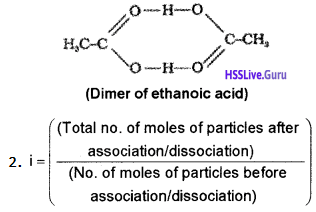Question 6.
The partial pressure of the gas in vapour phase is proportional to the mole fraction of the gas in the solution. This is the commonly used law for expressing the solubility of gas in liquid.

1. Name the law. Write its mathematical expression.
2. What are the factors affecting the solubility of a gas in a liquid? Explain.

1. Roult’s law.
For an ideal solution containing two volatile components A and B,
PA = P°A χA,
PB = P°B χB and
P[Total] = PA + PB = P°A χA + P°B χB

2. The factors affecting the solubility of a gas in a liquid:

• Nature of the gas and the liquid – Each gas has a characteristic solubility in a particular liquid at a particular temperature and pressure.
• Temperature – solubility of a gas in a liquid is an exothermic process. Hence according to Le Chatelier’s principle solubility of gases in liquids decreases with rise in temperature.
• Pressure – According to Henry’s law, solubility of a gas in a liquid is directly proportional to the pressure of the gas.

Question 7.
Concentration of solution may be expressed in different ways.

1. Mention any one of the concentration terms.
2. What are colligative properties?
3. Show that elevation in boiling point is a colligative property.

1. Molarity – It is the number of moles of the solute present in one litre of the solution.

2. Colligative properties are those properties which depends only on the number of solute particles.

3. ΔTb = Kbm
= $$\mathrm{k}_{\mathrm{b}} \frac{\mathrm{n}_{\mathrm{B}} \times 1000}{\mathrm{W}_{\mathrm{A}}}$$
i.e., ΔTb α nB i.e., elevation of boiling point depends on number of moles of solute. Hence, it is a colligative property.

### Plus Two Chemistry Solutions NCERT Questions and Answers

Question 1.
Concentrated nitric acid used in the laboratory work is 68% nitric by mass in aqueous solution. What should be the molarity of such a sample of the acid if the density of the solution is 1.504 g mL-1?Question 2.
Why does gases always tend to be less solube in liquids as the temperature is raised?
Dissolution of gases is an exothermic process. It is because of the fact this process involves decrease of entropy (ΔS < 0). Thus, increase of temperature tends to push the equilibrium,
Gas + Solvent $$\rightleftharpoons$$ Solution; ΔH = -ve
in the backward direction, thereby, supressing the dissolutionQuestion 3.
The vapour pressure of water is 12.3 kPa at 300 K. Calculate vapour pressure of 1 molal solution of a non-volatile solute in it.
H2O= 12.3 kPa
1000
In 1 molal solution, nsolute = 1; nH2O= $$\frac{1000}{18}$$ = 55.5
∴ χH2O = $$\frac{55.5}{55.5+1}$$
Vapour pressure of the solution, Ps = P°H2O × χH2O
= 0.982 × 12.3 = 12.08 kPa

Question 4.
Determine the osmotic pressure of a solution prepared by dissolving 25 mg of K2SO4 in 2 litre of water at 25°C, assuming that it is completely dissociated.
$$\frac{3 \times 25 \times 10^{-3} \mathrm{g} \times 0.0821 \mathrm{L} \mathrm{atm} \mathrm{K}^{-1} \mathrm{mol}^{-1} \times 298.15 \mathrm{K}}{174 \mathrm{g} \mathrm{mol}^{-1} \times 2 \mathrm{L}}$$